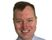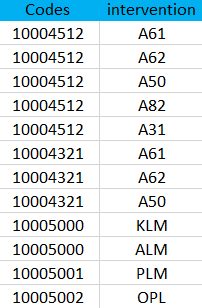cancel
Showing results for
Did you mean:Anonymous
Not applicable

## Dax measure / need help with a measure

Hi everyone,

I would like to do this measure :

calculate the number of interventions using the distinctcount function for which there is neither the "A61" nor the "A62" intervention, but also does not take into account other interventions including the co-response codes A61 AND A62 Here is the table. Table name: Table.

Another information: there are a lot of data like this.Thank you, My best regards.

2 ACCEPTED SOLUTIONSSuper User

I'm sorry, but I don't understand your question.

What do you mean by "calculate the number of interventions"?

Can you tell us what result you would expect the measure to return from the sample data you posted?Super User

@Anonymous wrote:

the result will be = 3

Ah ok, So you are counting distinct values from the Codes column provided that none of the interventions for that code are A61 or A62.

One was of doing this is a measure like the following:

```DCount Codes =
var _excludedCol1 = CALCULATETABLE(ALLSELECTED('Table'[Codes]), FILTER(ALL('Table'),'Table'[Intervention] IN {"A61","A62"}))
var _result = CALCULATE(DISTINCTCOUNT('Table'[Codes]), not 'Table'[Codes] in _excludedCol1)
return _result ```

I basically create the first variable as a list of Codes that do have one of A61 or A62, then I exclude these codes from the distinct count.

4 REPLIES 4Super User

I'm sorry, but I don't understand your question.

What do you mean by "calculate the number of interventions"?

Can you tell us what result you would expect the measure to return from the sample data you posted?Anonymous
Not applicable

Hi,

to be more preciseAnonymous
Not applicable

the result will be = 3Super User

@Anonymous wrote:

the result will be = 3

Ah ok, So you are counting distinct values from the Codes column provided that none of the interventions for that code are A61 or A62.

One was of doing this is a measure like the following:

```DCount Codes =
var _excludedCol1 = CALCULATETABLE(ALLSELECTED('Table'[Codes]), FILTER(ALL('Table'),'Table'[Intervention] IN {"A61","A62"}))
var _result = CALCULATE(DISTINCTCOUNT('Table'[Codes]), not 'Table'[Codes] in _excludedCol1)
return _result ```

I basically create the first variable as a list of Codes that do have one of A61 or A62, then I exclude these codes from the distinct count.Announcements#### Exclusive opportunity for Women!

Join us for a free, hands-on Microsoft workshop led by women trainers for women where you will learn how to build a Dashboard in a Day!#### Power Platform Conference-Power BI and Fabric Sessions

Join us Oct 1 - 6 in Las Vegas for the Microsoft Power Platform Conference.Top Solution Authors
Top Kudoed Authors
Users online (2,020)